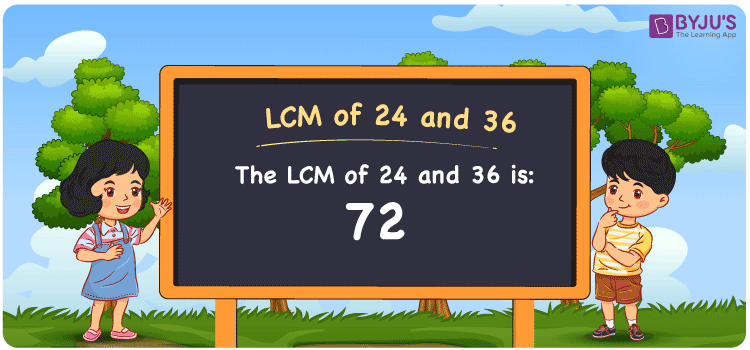Checkout JEE MAINS 2022 Question Paper Analysis : Checkout JEE MAINS 2022 Question Paper Analysis :

# LCM of 24 and 36

LCM of 24 and 36 is 72. In Maths, the LCM of any two numbers is the value which is evenly divisible by the given two numbers. Least common multiple of 24 and 36 is the smallest number we get among the common multiples. (24, 48, 72, 96, ….) and (36, 72, 108, 144,….) are the first few multiples of 24 and 36. The LCM can be found easily by using various methods like prime factorisation, division and by listing the multiples.

## What is LCM of 24 and 36?

The answer to this question is 72. The LCM of 24 and 36 using various methods is shown in this article for your reference. The LCM of two non-zero integers, 24 and 36, is the smallest positive integer 72 which is divisible by both 24 and 36 with no remainder.## How to Find LCM of 24 and 36?

LCM of 24 and 36 can be found using three methods:

• Prime Factorisation
• Division method
• Listing the multiples

### LCM of 24 and 36 Using Prime Factorisation Method

The prime factorisation of 24 and 36, respectively, is given by:

24 = 2 x 2 x 2 x 3 = 2³ x 3

36 = 2 x 2 x 3 x 3 = 2² x 3²

LCM (24, 36) = 72

### LCM of 24 and 36 Using Division Method

We’ll divide the numbers (24, 36) by their prime factors to get the LCM of 24 and 36 using the division method (preferably common). The LCM of 24 and 36 is calculated by multiplying these divisors.

 2 24 36 2 12 18 2 6 9 2 3 9 3 1 3 1 1

No further division can be done.

Hence, LCM (24, 36) = 72

### LCM of 24 and 36 Using Listing the Multiples

To calculate the LCM of 24 and 36 by listing out the common multiples, list the multiples as shown below

 Multiples of 24 Multiples of 36 24 36 48 72 72 108 96 144 120 180

LCM (24, 36) = 72

## Video Lesson on Applications of LCM## LCM of 24 and 36 Solved Examples

Find the LCM if the product of two numbers is 864 and the GCD is 12.

Solution:

It is given that

Product of two numbers = 864

GCD = 12

We know that

LCM x GCD = Product of two numbers

LCM = Product/GCD

LCM = 864/12

LCM = 72

Hence, the LCM is 72.

## Frequently Asked Questions on LCM of 24 and 36

### What is the LCM of 24 and 36?

The LCM of 24 and 36 is 72. To know the LCM, we first have to find the multiples of 24 and 36 and the smallest multiple which is exactly divisible by 24 and 36 has to be determined.

### List the methods used to find the LCM of 24 and 36.

The methods used to find the LCM of 24 and 36 are Prime Factorization Method, Division Method and Listing multiples.

### Find the GCF if the LCM of 24 and 36 is 72.

LCM x GCF = 36 x 24

Given,

LCM of 24 and 36 = 72

72 x GCF = 864

GCF = 864/72 = 12